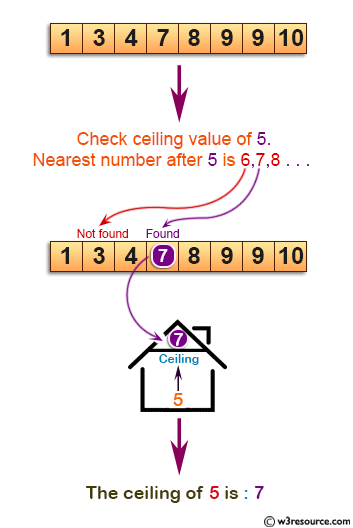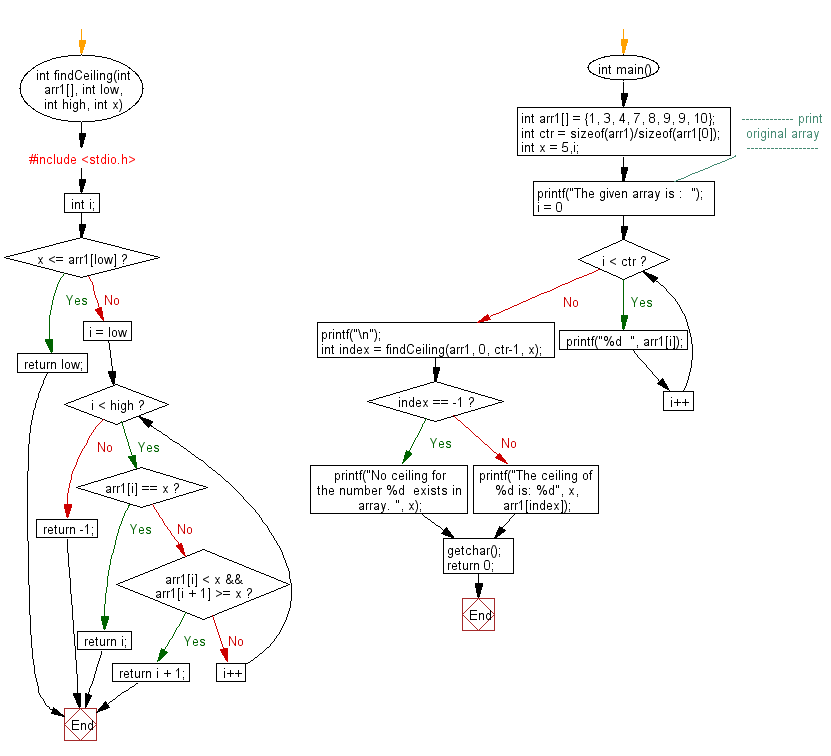﻿ C exercises: Find the ceiling in a sorted array - w3resource# C Exercises: Find the ceiling in a sorted array

## C Array: Exercise-40 with Solution

Write a program in C to find the ceiling in a sorted array.
N.B.: Given a sorted array in ascending order and a value x, the ceiling of x is the smallest element in array greater than or equal to x, and the floor is the greatest element smaller than or equal to x.

Pictorial Presentation:Sample Solution:

C Code:

``````#include <stdio.h>

int findCeiling(int arr1[], int low, int high, int x)
{
int i;
if (x <= arr1[low])
return low;
for (i = low; i < high; i++)
{
if (arr1[i] == x)
return i;

if (arr1[i] < x && arr1[i + 1] >= x)
return i + 1;
}
return -1;
}

int main()
{
int arr1[] = {1, 3, 4, 7, 8, 9, 9, 10};
int ctr = sizeof(arr1)/sizeof(arr1);
int x = 5,i;
//------------- print original array ------------------
printf("The given array is :  ");
for(i = 0; i < ctr; i++)
{
printf("%d  ", arr1[i]);
}
printf("\n");
//----------------------------------------------------
int index = findCeiling(arr1, 0, ctr-1, x);
if (index == -1)
printf("No ceiling for the number %d  exists in array. ", x);
else
printf("The ceiling of %d is: %d", x, arr1[index]);
getchar();
return 0;
}
```
```

Sample Output:

```The given array is :  1  3  4  7  8  9  9  10
The ceiling of 5 is: 7
```

Flowchart:C Programming Code Editor:

Improve this sample solution and post your code through Disqus.

What is the difficulty level of this exercise?

﻿